Search
Document Center

# Identify the most relevant poluttant for haze

Last Updated: May 14, 2020

## BackgroundAir pollution has become one of the top 10 issues that people are worried about. Air pollution, or haze, not only affects how people travel and entertain themselves, but also presents a hazard to public health. This example analyzes the weather data of Beijing collected in 2016 and finds that nitrogen dioxide was the most relevant pollutant for haze (PM 2.5).

Log on to Alibaba Cloud Machine Learning Platform for AI (PAI) Studio to create an air pollution haze prediction experiment by using a template.

## Dataset

Data source: This dataset was created based on the weather data of Beijing in 2016.

Air index data for each hour since January 1, 2016 was collected. The fields are as follows.

Field Definition Type
time Date, accurate to the day string
hour The hour of the data string
pm2 The PM2.5 index. string
pm10 The PM10 index. string
so2 The sulfur dioxide index. string
co The carbon monoxide index. string
no2 The carbon dioxide index. string

## Data exploration procedure

The experiment process is as follows.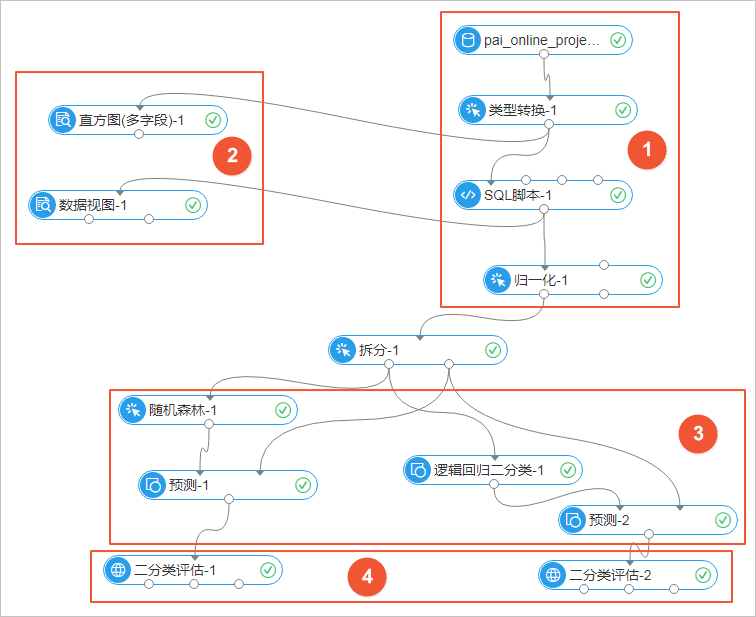The entire experiment is divided into four parts: data import and preprocessing (1 in the preceding figure), statistical analysis (2 in the preceding figure), model training and prediction (3 in the preceding figure), and model evaluation and analysis (4 in the preceding figure). The details are as follows.

#### 1. Data import and preprocessing

1. Data import
Click Data Source, select Create Table, and upload a .txt or .csv file.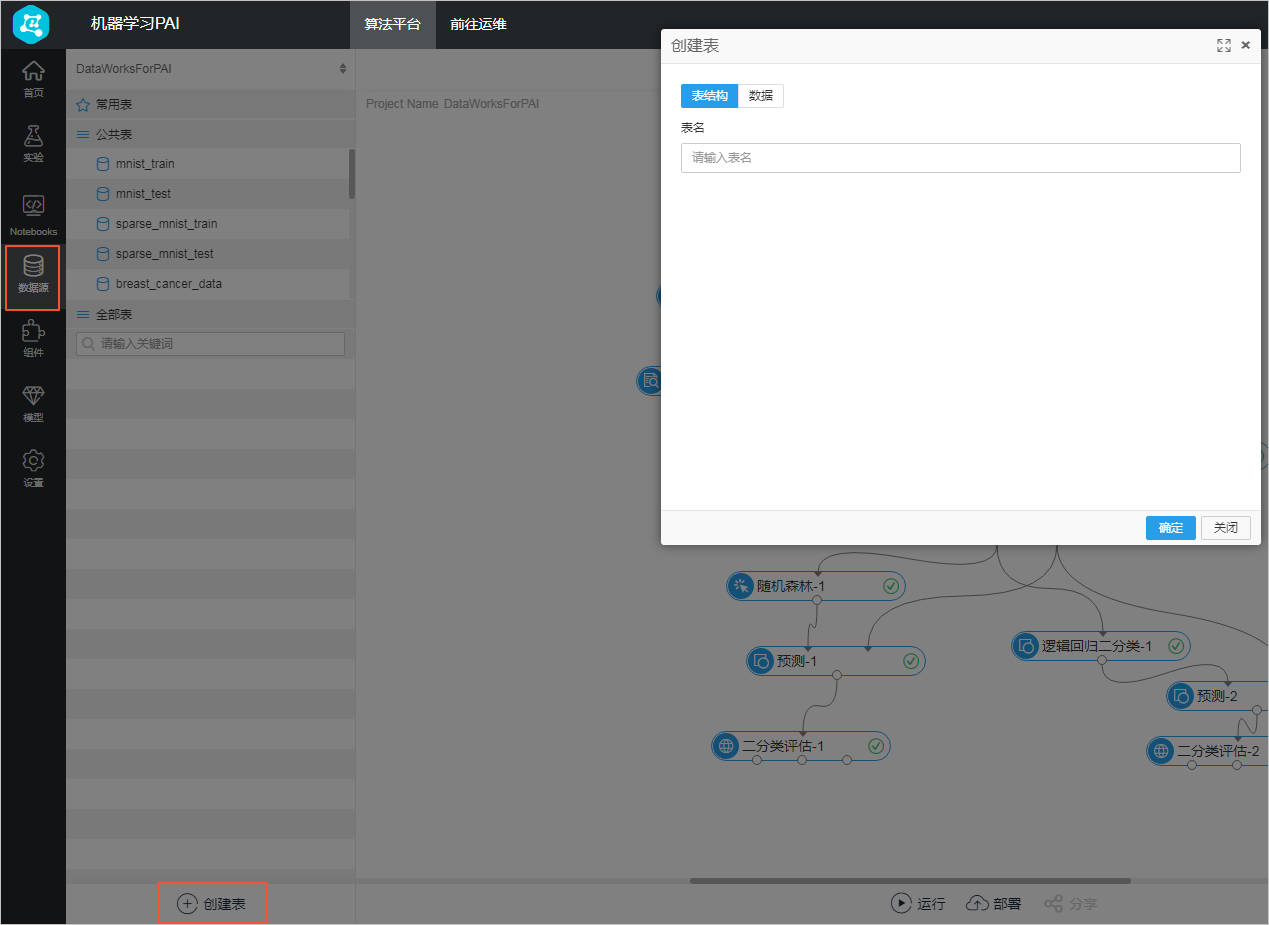After the data is imported, right-click the component and choose View Data from the shortcut menu. The result is as follows.1. Data preprocessing
Convert data of the string type to the double type through the Data Type Conversion component.
Convert the target column to a double type of 0 and 1 through the SQL Script component. In this experiment, “pm2” is listed as the target column. Values larger than 200 are marked as 1 for heavy haze, and values smaller than or equal to 200 are marked as 0. The SQL statement is as follows.
``select time,hour,(case when pm2>200 then 1 else 0 end),pm10,so2,co,no2 from \${t1};``
1. Normalization
Normalization aims to remove the dimension, that is, to unify the units of pollutants with different indexes.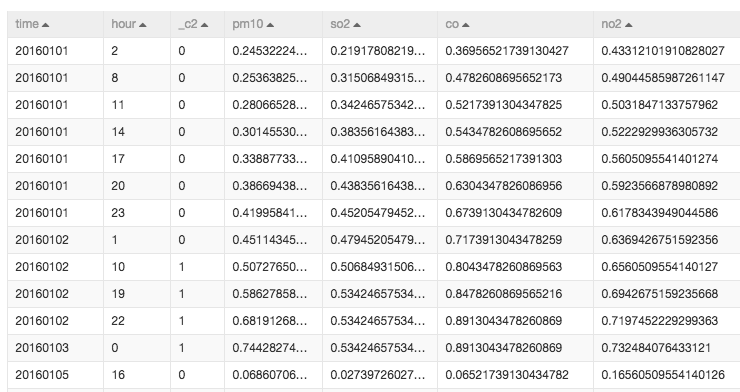#### 2. Statistical analysis

1. Histogram
The Histogram component allows you to view the distribution of the data in different intervals.
This experiment visually presents the distribution of data in each field. As shown in the following figure, taking PM2.5 (pm2) as an example, the most significant range of values is 11.74 to 15.61, with a total of 430 records.2. Data View
The Data View component allows you to view the impact of intervals with different metrics for the prediction results.
For example, seven instances with value 0 and nine instances with value 1 fall into the 112.33 to 113.9 interval. This indicates that when the nitrogen dioxide index is between 112.33 and 113.9, the probability of heavy haze is large. The entropy and Gini coefficient indicate the impact of this feature range on the target value (the impact on the aspect of information), and the larger the value, the greater the impact.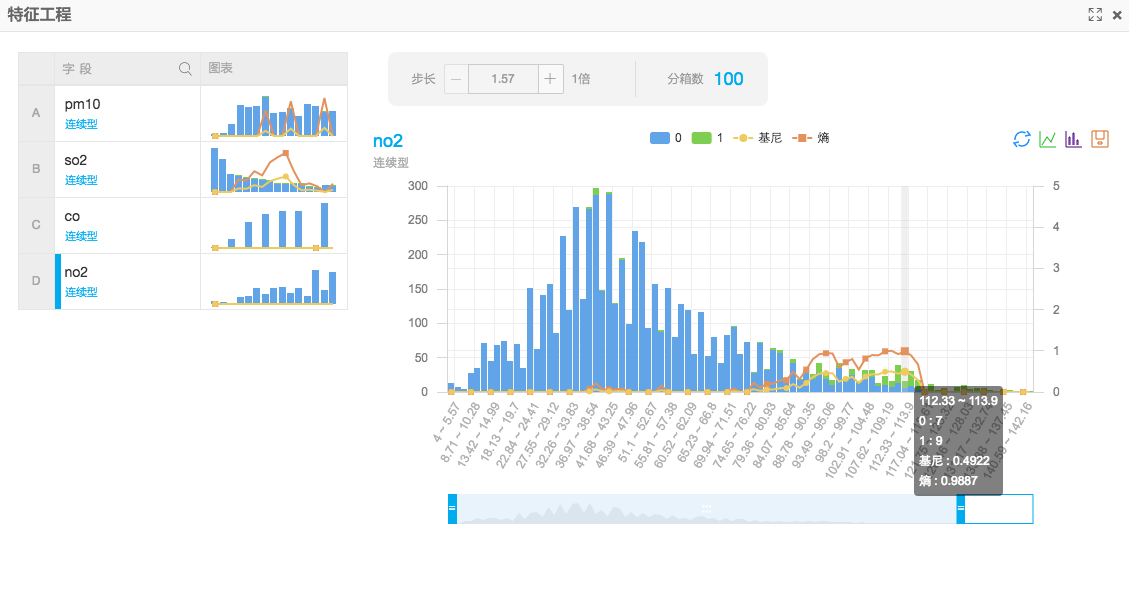#### 3. Model training and prediction

In this example, two different algorithms are used to predict and analyze the results: random forest and logistic regression.

##### Random forest

The dataset is split, in which 80% is used for model training, and 20% is used for prediction. In the left-side navigation pane of the console, click Models and select Saved Models. Right-click the model and choose Show Model from the shortcut menu. Then, the tree model of the random forest is visually shown as follows.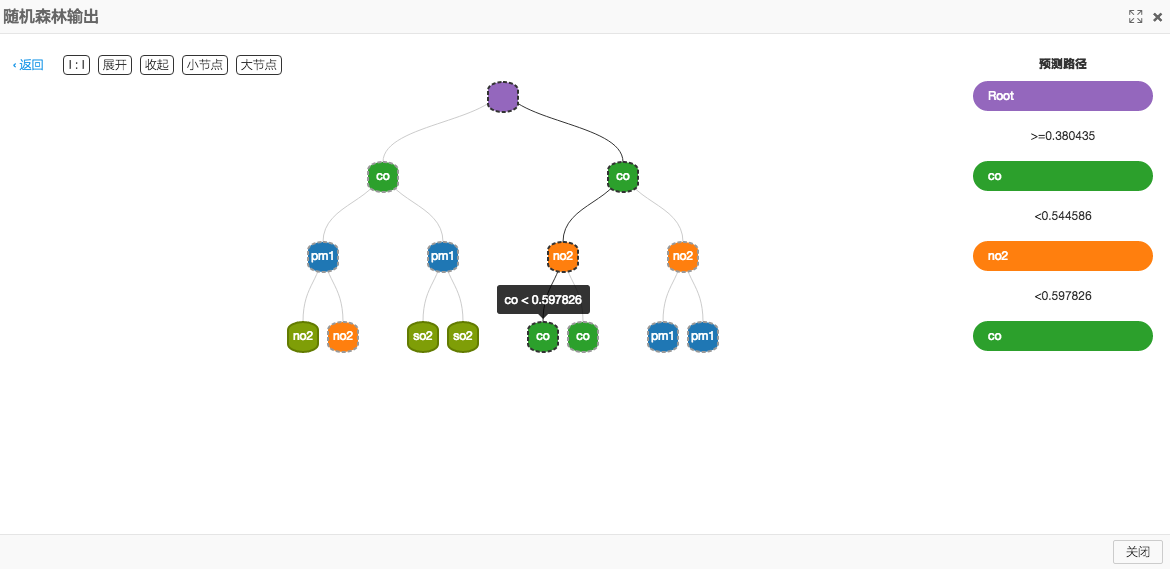The prediction result is as follows.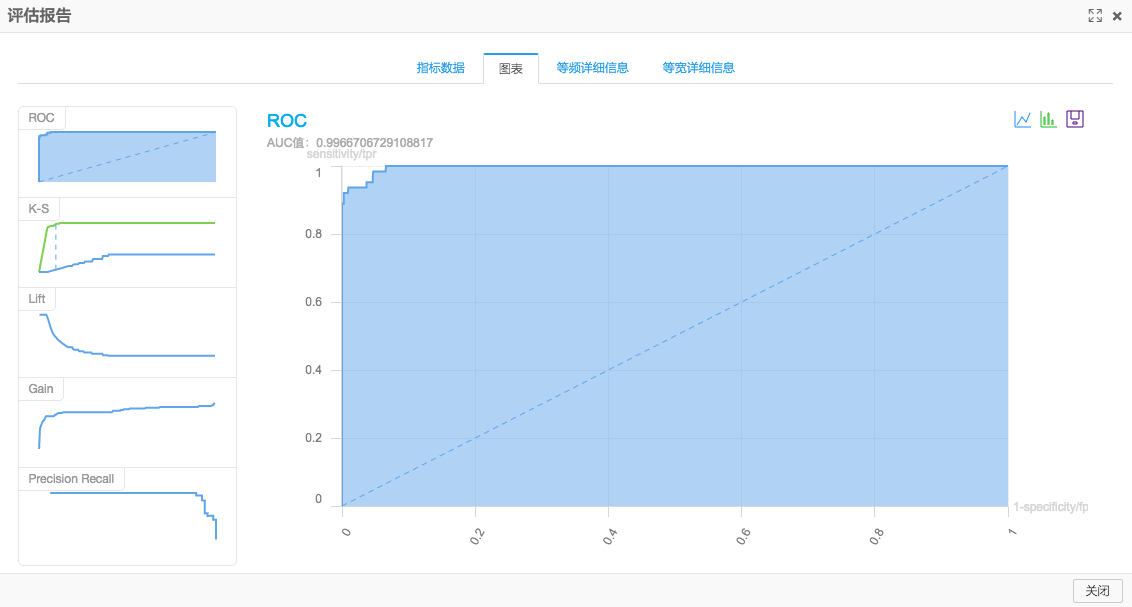The AUC in the preceding figure is 0.99, which indicates that with the weather index data used in this example, it can predict whether haze will occur, and the accuracy rate can reach more than 90%.

##### Logistic regression

A linear model can be obtained by training with the logistic regression algorithm, as shown in the following figure.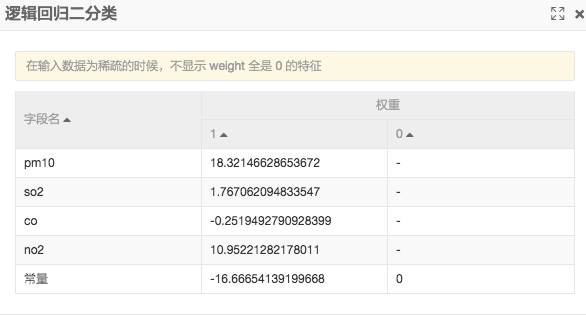The prediction result is as follows.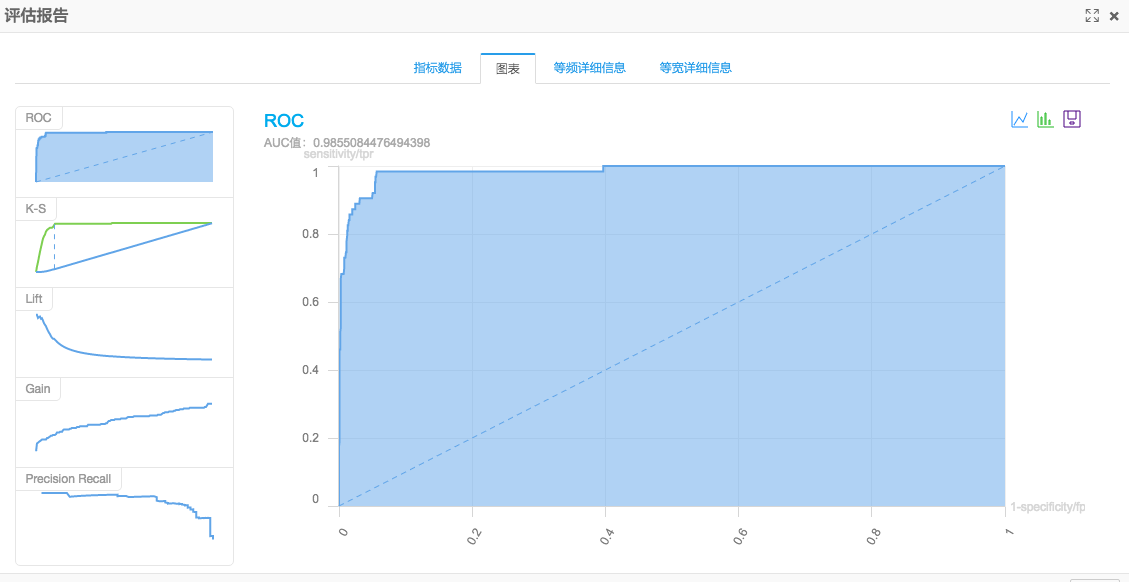The result shows that the AUC is 0.98, which is a little lower than the prediction accuracy based on random forest. If you exclude the impact of parameter adjustments, the two prediction results show that random forest trains your model better than logistic regression.

## Model evaluation and analysis

Based on the preceding model and prediction results, the air index with the greatest impact on PM2.5 is analyzed.

The logistic regression model generated is shown in the following figure.The impact on the result is proportional to the model coefficient of the logistic regression algorithm after normalized computing. The coefficient symbol is positive for positive correlation and negative for negative correlation. In the preceding figure, pm10 and no2 have the greatest positive coefficients.

• The difference between pm10 and pm2 is the size of fine particles. Therefore, the impact of pm10 is not considered.
• NO2 (nitrogen dioxide) has the greatest impact on PM2.5. You can check the relevant documents to find out which factors will cause a large amount of nitrogen dioxide emissions and identify the major factors that affect PM2.5.
The article Source of Nitrogen Dioxide from the Internet indicates that nitrogen dioxide mainly comes from vehicle exhaust.

## References

You can log on to Alibaba Cloud PAI to experience this product and go to Yunqi Community to discuss it with us.